Mobile QR Code1. (The School of Computer Science and Engineering, Kyungpook National University, Daegu 41566, Korea)
2. (Department of Computer Engineering, Pukyong National University, Busan 48513, Korea)

Approximate computing, approximate adder, energy efficiency, machine learning

## I. INTRODUCTION

Nowadays, data is being produced anywhere and anytime at an alarming rate, and energy consumption for processing the data also increases very quickly. Also, with the rapid growth of the internet, various types of battery-dependent smart devices have become more and more common. These devices are running many applications that process vast amounts of data that are computationally demanding for machine learning and multimedia (e.g., audio, image, video) processing [1-4]. As the use of battery-dependent devices increases and the energy consumed by them continues to grow, today’s computing technologies face the challenge of low-power and energy-efficient system design. The key observation of these applications is that although an insignificant error occurs in processing the data, it is difficult for human beings to recognize if an error occurs due to the human’s cognitive ability. For example, when the quality of the image is marginally degraded (e.g., salt and pepper noise), the human may still be able to understand what the image represents. Therefore, applications that process these data related to the human sense allow for some degree of error in their data processing. This leads to a power and energy reduction in the operations by sacrificing the marginal accuracy, which is known to approximate computing that trades power and energy for accuracy [5,6].

This paper proposes a novel approximate adder design based on the split architecture using an efficient carry speculation technique and a truncation scheme. While our preliminary work has been presented in , in this work, we improve our adder architecture and performance by systematically analyzing it and addressing several key issues. Our earlier adder in  has a good accuracy performance, while it shows a poor hardware efficiency and no scalability of the design. Hence, we propose a scalable approximate adder design by introducing a nonzero truncation scheme. Additionally, we perform a mathematical analysis to characterize the design and extensively compare the proposed adder with others to prove the competitivity of the proposed design. The main contributions of this paper are as follows:

• We propose a novel approximate adder design based on modified FA and nonzero constant truncation for good tradeoff between the accuracy and hardware.

• We systematically examine the hardware and accuracy of the proposed adder both by mathematical analysis and experimental validation and compare it with other ten adders thoroughly.

• We demonstrate the efficacy of the proposed adder in real-world applications by adopting various adders in machine learning and digital image processing.

## II. RELATED WORKS

A significant number of approximate adder has been presented to reduce power and energy consumption of digital systems. Fig. 1 illustrates the operation of the approximate mirror adder 5 (AMA5), one of the mirror adders in . The n-bit AMA5 consists of a k-bit accurate part that includes a precise adder and an (n-k)-bit inaccurate adder part where the adder outputs one of the input pair, and the MSB of the other pair is propagated as a carry prediction signal for the precise adder. This design does not require any computation between two input pairs in the inaccurate part, leading to good hardware efficiency. Fig. 2 demonstrates the block diagram of the lower-part OR adder (LOA) . Its inaccurate adder part outputs the OR computation results of two input pairs. In addition, the LOA performs an AND-based carry prediction from the MSB input pair of the inaccurate adder part to the precise adder to improve an overall accuracy. Some modifications of the LOA have been proposed to further enhance the performance. The optimized lower part constant-OR adder (OLOCA) sets some output bits of the inaccurate part to a constant 1'' rather than the result of OR operations . Similar to the OLOCA, the lower-part OR truncation adder (LOTA) has a part that outputs the OR operation results and a part that outputs 1'' . However, instead of AND-based carry prediction, the LOTA performs carry prediction similarly to the AMA5. The error tolerant adder I (ETAI) performs a modified XOR operation in the inaccurate part . Unlike the AMA5 and LOA, it does not have any carry prediction scheme. This slightly degrades the accuracy while improving the delay and power consumption. The simplified ETAI (SETA), which is a variant of the ETAI, was presented to improve the hardware performance of the ETAI . While the ETAI checks all input pairs in the inaccurate part to examine if the values of an input pair are both 1'', the SETA only checks a specific position of a pair. This makes the SETA provide better hardware performance than the ETAI without significant accuracy degradation. The error-tolerant constant adder (ETCA) is also a variant of the ETAI and sets some output values to 1'' , like the OLOCA. The energy quality scalable adder (EQSA) can dynamically change the design as needed in consideration of the trade-off between energy and accuracy, and it adopts a structure that sets the output to 1'' regardless of the input in the inaccurate part . In , the hardware optimized and having a near-normal error distribution adder (HOAANED) that optimizes hardware performance and improves error characteristics of an approximate adder has been proposed.

##### Fig. 1. Operation of the AMA5.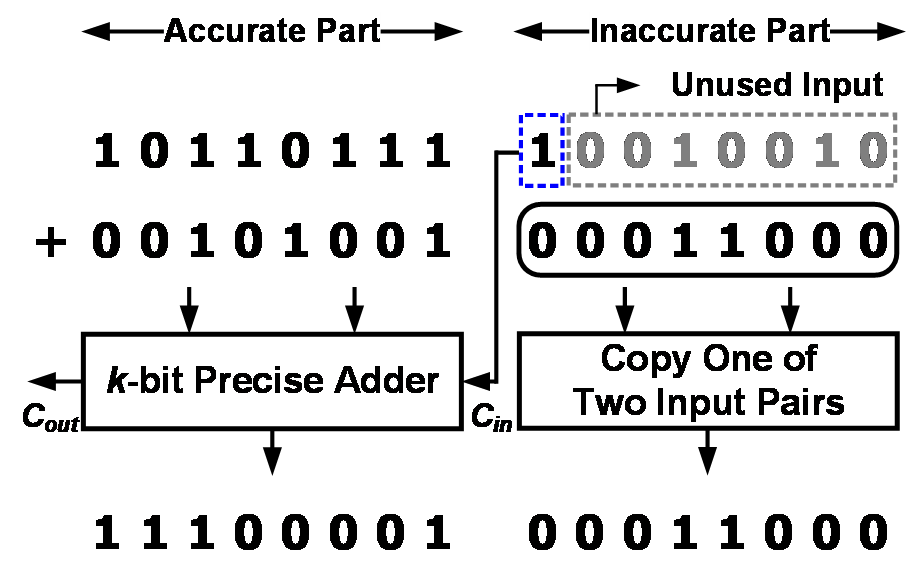##### Fig. 2. Block diagram of the LOA.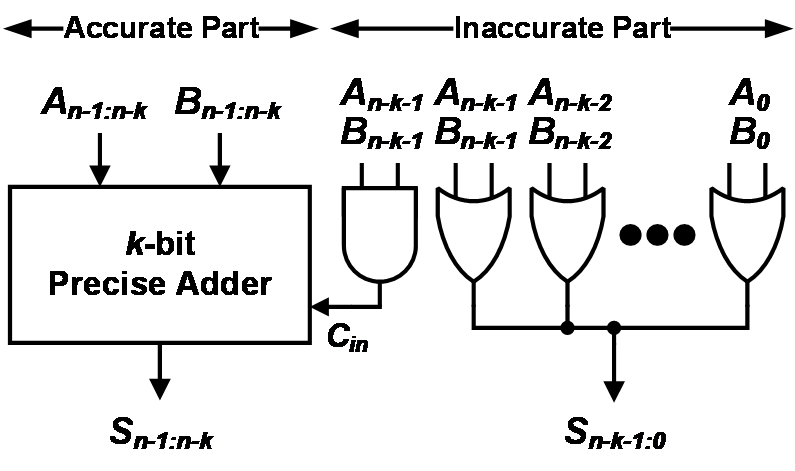### 1. Proposed Approximate Adder Architecture

Fig. 3 demonstrates the block diagram of the proposed approximate adder, termed AND-based carry prediction and constant truncation approximate adder (AC$^{2}$A). We denote a pair of n-bit inputs and an n-bit output of the adder as A$_{n-1\colon 0}$, B$_{n-1\colon 0}$, and S$_{n-1\colon 0}$, respectively, and (i)$^{th}$ least significant bit (LSB) of the A, B, and S as A$_{i}$, B$_{i}$, and S$_{i}$, respectively. The n-bit adder is divided into a k-bit accurate and an (n-k)-bit inaccurate part. To ensure an overall accuracy, the k-bit precise adder is placed in the upper position containing the MSBs since it significantly impacts on the overall addition result. Note that any of the conventional adders (e.g., RCA and CLA) can be used for the precise one. Also, the proposed adder adopts an AND-based carry prediction scheme from the inaccurate part to the accurate part to improve the accuracy (see C$_{in}$). The inaccurate part is divided into two parts: 1) the modified FA part that includes an AND-based carry and an OR-based sum generation logics, which perform the approximate addition for A$_{n-k-1\colon l}$ and B$_{n-k-1\colon l}$ and 2) the constant part, which sets each output bit to 1'' regardless of the corresponding input pair for the lowest l-bit containing LSBs. In the former part, the summation is basically conducted by ORing of the two input bits A$_{i}$ and B$_{i}$ and the carry predicted from the previous bit position C$_{i-1}$ and thus its Boolean equation becomes S$_{i}$= A$_{i}$+ B$_{i}$+ C$_{i-1}$. While the earlier works do not include bit-by-bit carry speculation logic [12-20], the proposed design offers the AND-based carry signal C$_{i}$= A$_{i}$· B$_{i}$ for each bit position to improve overall accuracy performance. Here, it is important to note that the MSB position of the inaccurate part (i.e., (n-k-1)$^{th}$ bit position) exploits XOR instead of OR to approximately add the two input A$_{n-k-1}$ and B$_{n-k-1}$ since the XOR forms the exact half adder structure with an AND gate, resulting in a higher accuracy. The OR gate is relatively cheaper than the XOR in terms of hardware cost, but the XOR and OR gate yield the same output except for the case of A$_{i}$ = B$_{i}$ = 1 out of the four possible input combinations of the input pair. Therefore, to reduce hardware cost without any significant accuracy loss, we leverage the OR gate to produce the approximate summation of A$_{n-k-2\colon l}$ and B$_{n-k-2\colon l}$. In the latter part, the hardware cost reduction can be expected by simply setting the part that has a relatively small effect on the result of the addition (i.e., LSBs) to 1'' without using any logic gate. Particularly, it reduces the error distance by setting the output to 1'' rather than 0'' because the carry prediction from the inaccurate part to the accurate part (i.e., C$_{in}$) may not be correct compared to the precise adder due to cut of the carry chain from the LSB, and the overall approximate summation could become smaller than the correct one. It is worth noting that the length of the constant part can be adjusted to obtain the good tradeoff between the computation accuracy and hardware efficiency. For example, a longer length of the constant part will improve the hardware efficiency but degrade the overall accuracy performance.

##### Fig. 3. Block diagram of the proposed approximate adder.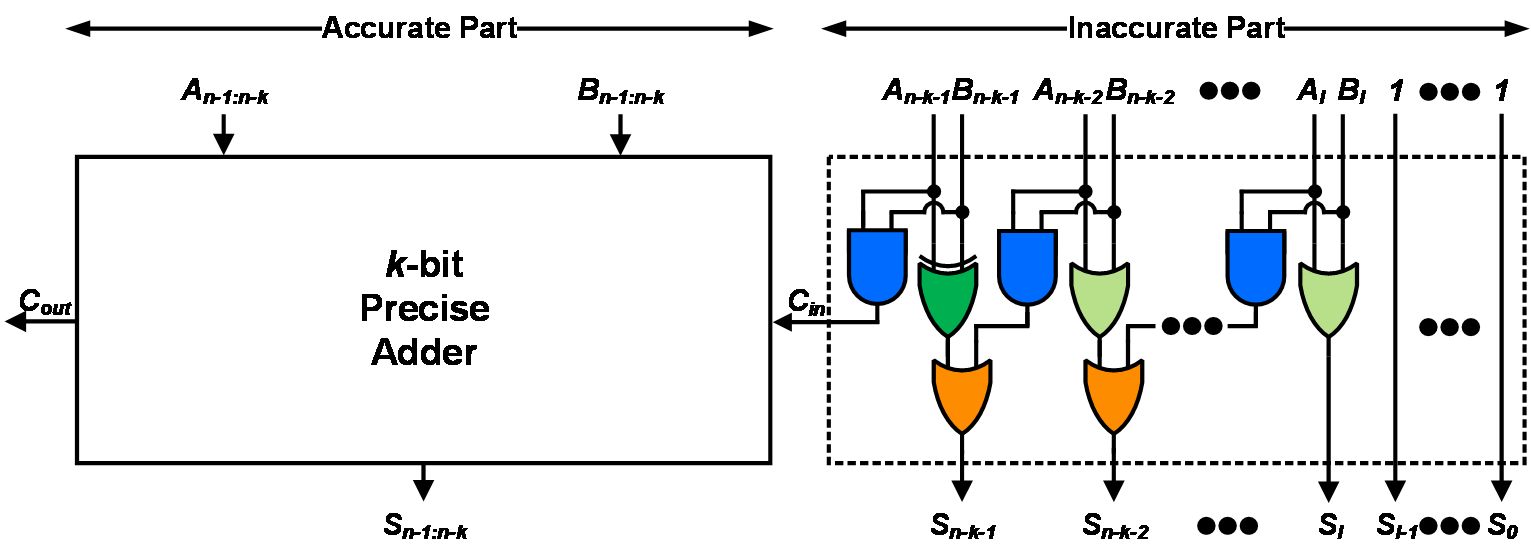### 2. Error Rate Analysis

The error rate is one of the most important metrics when evaluating the accuracy of approximate adders. In this paper, we analyzed the case where errors occur by deriving a formula for the error rate of the proposed adder. We assume that two input operands A and B are bitwise independent. To derive the error rate in a simplistic way, we first take into account the input cases where no error is introduced. Then, we can obtain the error rate by the probability of a complementary event of the cases. Note that the analysis of the accurate part is excluded here since the exact adder does not generate any error. From (n-k-2)$^{th}$ bit to (l)$^{th}$ bit with OR gates applied instead of XOR gates, if each input pair of the bit position from (n-k-2)$^{th}$ to (l)$^{th}$ is both 1'', then an error occurs because the corresponding output bit becomes 1'' due to the OR operation. In other words, the output value is always correct when the input pair is not both 1''. In addition, if A$_{n-k-2}$ ${\neq1}$ and B$_{n-k-2}$ ${\neq1}$ , the carry to $S_{n-k-1}$(i.e., C$_{n-k-2}$) is not propagated. Then, the error at the (n-k-1)$^{th}$ bit position can be excluded for the error rate analysis since this bit position forms a half adder structure. For the l-bit constant part, no error occurs when each bit of the input pair is different from each other. In other words, when each bit of the input pair is equal (i.e., A$_{i}$ = B$_{i}$), an error occurs with the corresponding bit output of 1'' although the correct sum is 0''. In short, the proposed adder always produces correct output under the following two conditions: 1) the input pair is A$_{i}$ ${\neq1}$ and B$_{i}$ ${\neq1}$ where n-k-2 ${\leq}$ i ${\leq}$ l and 2) each bit of the input pair is different from each other in the position from (l-1)$^{th}$ to (0)$^{th}$ bit. Considering both, we can define an event E$_{correct}$ that the adder yields correct additions by:

##### (1)
$E_{correct}=\prod _{i=l}^{n-k-2}\left(\overline{A_{i}B_{i}}\right)\cdot \prod _{i=0}^{l-1}\left(A_{i}\overline{B_{i}}+\overline{A_{i}}B_{i}\right).$

Then, the error rate of the proposed adder can be derived by the complementary probability of the event as follows:

##### (2)
$\mathrm{ER}\left(n,k,l\right)=1-\mathrm{P}\left(E_{correct}\right)=1-\left(\frac{3}{4}\right)^{n-k-l-1}\cdot \left(\frac{1}{2}\right)^{l}.$

To verify the adder’s error rate analysis, we conducted a simulation to obtain the error rate values by applying 10 million uniformly distributed random input pairs and compare them with the derived equation. Here, the lengths of the entire adder n and the precise one were set to 16 and 8, respectively. Also, the size of the constant part l was swept from 1 to 7. Table 1 shows the error rate values obtained by the simulation and formula. As can be seen, the derived error rate well matches the simulation results over the various parameter values.

##### Table 1. Simulated and calculated error rates with various lengths of constant part
 l Calculated (%) Simulated (%) Difference 1 91.10 91.10 - 2 94.07 94.07 - 3 96.05 96.04 0.01 4 97.36 97.36 - 5 98.24 98.24 - 6 98.83 98.83 - 7 99.22 99.22 -

## IV. EXPERIMENTAL RESULTS

To evaluate the performance of the proposed adder in terms of the hardware performance and computation accuracy, we adopt a 16-bit adder and configure it by setting the size of the accurate part and inaccurate part to both 8 bits (i.e., n=16, k=8). Here, it is noteworthy that earlier works suggested that 7-bit to 9-bit sizes would be suitable for the inaccurate part, and a 16-bit adder is commonly used in these applications to achieve a good tradeoff between accuracy and power savings for practical applications such as image processing and machine learning, [12,28]. Therefore, we chose the design parameter n=16 and k=8. Particularly, two different constant part’s lengths of 0 and 4 (i.e., l=0 and l=4) are considered to examine the tradeoff of the accuracy and hardware according to the parameter l. We also take into account ten existing adders for performance comparison. We apply the same design parameter values to these adders. Here, the proposed adder structures according to l are represented by AC$^{2}$A (l=0) and AC$^{2}$A (l=4), respectively. Also, an RCA is adopted as the precise adder of the accurate part. The summary of the hardware and accuracy performance of the proposed and existing adders is shown in Table 2.

##### Table 2. Performance summary of various adders
 Design Area (μm2) Delay (ps) Power (μW) Energy (fJ) Error Rate (%) MED MRED (10-4) NMED (10-4) RCA 196.13 1833 59.94 109.85 - - - - CLA 302.08 735 66.93 49.2 - - - - AMA5  101.77 916 30.91 28.30 99.61 64.00 13.52 4.883 LOA  121.60 920 34.90 32.12 89.99 47.86 10.08 3.652 OLOCA  108.44 920 32.34 29.76 99.12 51.98 10.95 3.966 ETAI  132.71 897 34.02 30.50 89.99 51.18 10.74 3.905 SETA  119.96 897 32.08 28.76 89.99 55.81 11.72 4.258 ETCA  114.35 897 31.17 27.94 98.02 51.87 10.89 3.957 LOTA  104.00 916 31.44 28.79 99.80 66.55 14.08 5.077 EQSA  247.15 916 65.03 59.55 99.61 85.31 18.06 6.509 HOAANED  114.59 926 33.37 30.90 98.83 32.00 6.75 2.441 AC2A (l=0) 143.68 920 38.29 35.23 86.66 26.15 5.51 1.995 AC2A (l=4) 126.33 920 35.35 32.53 97.36 26.68 5.62 2.040

### 1. Hardware Performance Analysis

For hardware performance analysis, all twelve adders in Table 2 were designed in Verilog HDL and synthesized with a 32-nm CMOS technology. As metrics of hardware performance evaluation, area, delay, power, and energy, which is the product of power and delay, were extracted. The RCA shows the largest area, the longest delay, and the largest power consumption due to the long carry chain from the LSB to the MSB by the FAs. The CLA has a quite shorter delay than the RCA thanks to the carry look-ahead generator while it occupies a larger area because its carry generator requires a considerable number of logic gates. The CLA consumes less energy than the RCA due to its significantly shorter delay than the RCA’s despite its marginally larger power consumption. The AMA5 and LOA predict the carry signal by one of the input pair and the AND operation result of the input pair, respectively. Therefore, the AMA5 goes through one logic gate less than the LOA, resulting in a marginally shorter delay than the LOA. The LOA, OLOCA, and AC$^{2}$A (l=0) show the same delay since they utilize AND-based carry prediction. The OLOCA demonstrates a smaller area and less power consumption than the LOA. Its energy is also smaller than that of the LOA because some output bits are set to 1'' regardless of the input. The LOTA, which has a simpler structure than the OLOCA, shows superior performance in area and power consumption compared to the OLOCA. The ETAI has a shorter delay than the LOA due to a lack of carry prediction logic. The ETAI’s variants, such as the SETA and ETCA, also have the same delay as the ETAI. The SETA and ETCA, which are simplified versions of the ETAI, have better area, power, and energy performance than the ETAI. The EQSA has a delay that equals to the AMA5 since they perform carry prediction similarly. However, the EQSA has a larger area than the RCA due to its relatively complicated structure to adjust the computation accuracy dynamically according to the control signal. The HOAANED predicts a carry signal based on AND operations but has a longer delay than the LOA because the signal is also applied to the comparator of the inaccurate adder part, which leads to a larger fanout. The AC$^{2}$A (l=4) has the same delay as the LOA because it predicts a carry signal based on AND operation. In order to improve the hardware performance, the proposed adder adopts the nonzero constant truncation scheme. Therefore, the AC$^{2}$A (l=4) has a smaller area and less power consumption than the AC$^{2}$A (l=0). Specifically, the area and power of AC$^{2}$A (l=4) are reduced by 12% and 8%, respectively, compared to the AC$^{2}$A (l=0). The two designs have the same AND-based carry signal prediction, so they have the identical delay, but the AC$^{2}$A (l=4) reduces the energy consumption by 8% more than that of the AC$^{2}$A (l=0). Moreover, the proposed AC$^{2}$A (l=4) can reduce the area, power, and energy by 48.9%, 45.6%, and 45.4%, respectively, compared to the EQSA.

### 2. Accuracy Analysis

As the accuracy evaluation metrics, error rate, mean error distance (MED), mean relative error distance (MRED), and normalized mean error distance (NMED) were obtained by a software-based simulation using 10$^{7}$ uniformly distributed random input pairs, and these metrics are defined by the following equations:

##### (3)
\begin{align} MED&=\frac{1}{n}ED_{i}, \end{align}
##### (4)
\begin{align} MRED&=\frac{1}{n}\sum _{i=1}^{n}\left| \frac{ED_{i}}{S_{i,accurate}}\right| , \end{align}
##### (5)
\begin{align} NMED&=\frac{MED}{D}, \end{align}

where n is the number of inputs, ED$_{i}$ is the error distance for the i$^{th}$ item of input data, S$_{i}$ is the accurate output for the i$^{th}$ item of input data, and D is the maximum output of the accurate design . The AMA5, which outputs one of the input pair as a summation result, lags behind in terms of accuracy compared to the LOA, OLOCA, ETAI, and SETA that adopt OR or modified XOR operations. Also, the LOTA has similar accuracy characteristics to the AMA5. Since the OLOCA is a design that improves the hardware performance of the LOA by exploiting the truncation scheme, it shows a marginally lower accuracy performance than the LOA in terms of MED, MRED, and NMED. The proposed designs AC$^{2}$A (l=0) and AC$^{2}$A (l=4) offer two of the most accurate approximate adders in terms of the error rate, MED, MRED, and NMED and, as expected, the AC$^{2}$A (l=0) shows slightly better than the AC$^{2}$A (l=4) in these metrics. In short, the proposed AC$^{2}$A (l=0) demonstrates the best accuracy performance in all the error metrics and has a very competitive accuracy performance among the adders considered here.

### 3. Joint Metric Analysis

In order to observe the tradeoff between hardware performance and accuracy collectively, we consider a joint metric. Here, we adopt the energy-MRED product obtained by multiplying the energy representing hardware performance by the MRED representing accuracy one. The energy-MRED product values were normalized based on the LOA and are shown in Fig. 4. Note that the smaller the value, the better the accuracy compared to the energy consumed by the adder. The EQSA shows the largest energy-MRED product because both the energy and MRED of the EQSA are the largest compared to other approximate adders (see Table 2). The LOTA shows the second largest energy-MRED product because its MRED is the second largest value, although its energy is above average. The proposed two adder designs show the top two energy-MRED performance among the adders, and the AC$^{2}$A (l=4) is the best. Specifically, the product value of the AC$^{2}$A (l=4) is 83% smaller than that of the EQSA. Therefore, considering both energy consumption and accuracy, the proposed adder AC$^{2}$A (l=4) has the best performance.

##### Fig. 4. Normalized energy-MRED products of various approximate adders.}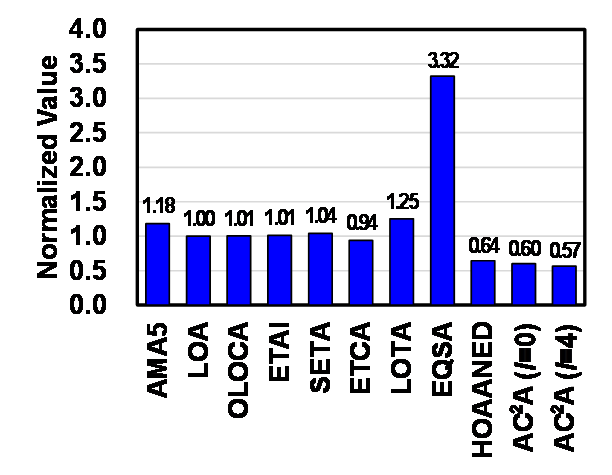## V. APPLICATIONS OF APPROXIMATE ADDERS

To examine that the proposed adder can produce good results in the practical applications, its performance was evaluated and compared with the other adders in machine learning and image processing applications. Specifically, we considered k-means clustering and Gaussian filtering.

### 1. Machine Learning

K-means clustering is an unsupervised learning used for clustering, such as image classification, and is one of the most widely used machine learning applications. The purpose of k-means clustering is to find similarities in the given data and divide them into k clusters. The addition is heavily used in k-means clustering, and we replace the accurate addition with the approximate ones. The constant k, which means the number of clusters, was set to 5 in our experiment. The performance of k-means clustering can be expressed as the within-cluster sum of squares (WCSS). The WCSS means the distance of data belonging to the cluster from the center of each cluster, and the shorter the distance, the better the clustering. Fig. 5 shows the visualized results of k-means clustering with the accurate and approximate adders, and the WCSS value of the corresponding adder is indicated next to the name of each adder. While the proposed AC$^{2}$A (l=4) demonstrates the best clustering performance in terms of WCSS, which means that its output is closest to the one by the error-free adder, the HOAANED and AC$^{2}$A (l=0) have similar WCSS values to the AC$^{2}$A (l=4). The AMA5, ETAI, ETCA, and EQSA are some of the poorest clustering performances among the adders, and the LOA, OLOCA, SETA, and LOTA are in-between. Particularly, the WCSS by the AC$^{2}$A (l=4) is 56% smaller than that by the ETAI. This proves that the proposed adder is well suitable for the machine learning application.

##### Fig. 5. Output images of k-means clustering using various adders.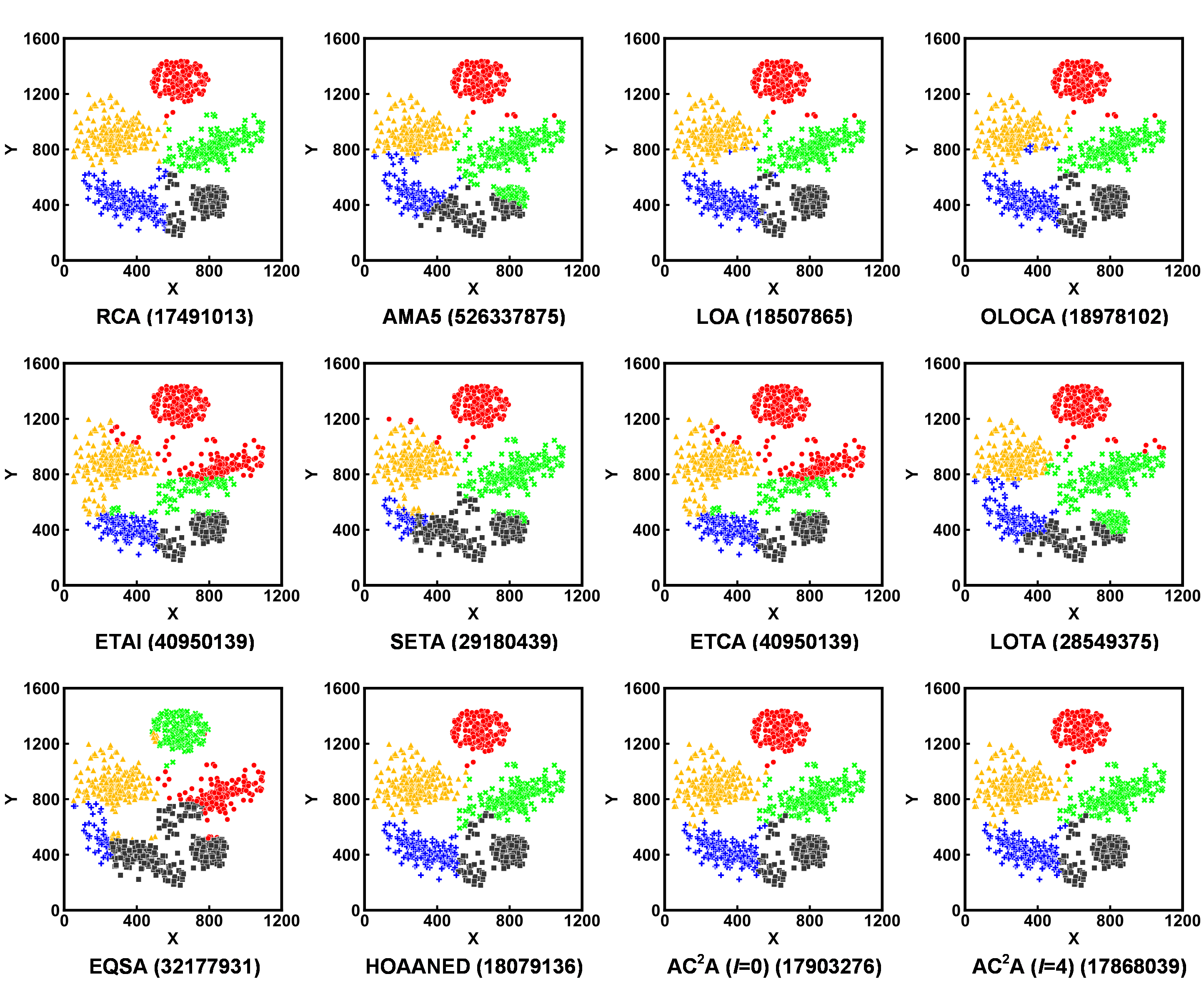### 2. Digital Image Processing

To demonstrate that the proposed adder is applicable for image processing applications, the Gaussian filtering was performed using various adders. Specifically, we used a 7${\times}$7 Gaussian filter in . This application also mainly utilizes the addition, which can be replaced by the approximate counterparts. The performance of the filtering can be represented by the Peak Signal-to-Noise Ratio (PSNR). Fig. 6 shows the output images of Gaussian filtering, and the PSNR value of the corresponding adder is indicated next to the name of each adder. Note that the PSNR was calculated against the image produced by the error-free adder RCA. The LOA and its variant OLOCA produce the images with the same PSNR value. The ETAI, its variants (SETA and ETCA), and EQSA are also the same. The AMA5 is in-between them. The AC$^{2}$A (l=4) and AC$^{2}$A (l=0) produce the images with the same PSNR value, which is the best value that exceeds 40 dB. This means that the proposed adders yield the output images closest to the one produced by the RCA. Therefore, we can expect the processing quality to be similar to those using the error-free adders with significantly reduced hardware resource consumption.

##### Fig. 6. Output images of Gaussian filtering using various adders.## VI. CONCLUSIONS

In this paper, we proposed an approximate adder design based on the modified FA and nonzero truncation scheme. The proposed adder showed the better accuracy and hardware performance compared to the other approximate adders considered in this paper. Specifically, the AC$^{2}$A (l=4) reduced MED and MRED by 44% compared to the LOA. In terms of hardware, the AC$^{2}$A (l=4) improved area, power, and energy by 48.9%, 45.6%, and 45.4%, respectively, compared to the EQSA. Considering both accuracy and hardware performance, the proposed adder showed the best result, specifically 83% better than the EQSA. Moreover, the proposed adder was adopted in the real-world applications, particularly, k-means clustering and Gaussian filtering, and showed the best processing quality compared to the other adders. This confirmed that it can reduce energy consumption without significant accuracy degradation while similar output quality to that by the error-free adder. Hence, excellent hardware and accurate performance can be expected when the proposed design is employed in various error-tolerant applications, such as machine learning and multimedia processing.

## ACKNOWLEDGMENTS

This work was supported in part by Institute of Information & communications Technology Planning & Evaluation (IITP) grant funded by the Korea government (MSIT) (No. 2021-0-00310, Development of SW Framework for Server to Improve AI Training/Inference Efficiency) and in part by the Basic Science Research Program through National Research Foundation of Korea (NRF) funded by the Ministry of Education (NRF-2019R1I1A3A01061266).

## References

1
J. H. Kim, C. Kim, K. Kim, J. Lee. H.-J. Yoo, and J.-Y. Kim, “An Ultra-low-power Mixed-mode Face Recognition Processor for Always-on User Authenication in Mobile Device,” IEIE Journal of Semiconductor Technology and Science, Vol. 20, No. 6, pp. 499-509, Dec., 2020.2
S. Ryu, “Review and Analysis of Variable Bit-precision MAC Microarchitectures for Energy-efficient AI Computation,” IEIE Journal of Semiconductor Technology and Science, Vol. 22, No. 5, pp. 353-360, Oct., 2022.3
J. Koo, J. Kim, S. Ryu, C. Kim, J.-J. Kim, “Area-efficient Transposable Crossbar Synapse Memory Using 6T SRAM Bit Cell for Fast Online Learning of Neuromorphic Processors,” IEIE Journal of Semiconductor Technology and Science, Vol. 20, No. 2, pp. 195-203, Apr., 2020.4
W. Shin and N. Baek, “Optimizing Ultra High-resolution Video Processing on Mobile Architecture with Massively Parallel Processing,” IEIE Transactions on Smart Processing and Computing, Vol. 10, No. 2, pp. 84-89, Apr., 2021.5
T. Moreau, A. Sampson, and L. Ceze, “Approximate Computing: Making Mobile Systems More Efficient,” IEEE Pervasive Computing, Vol. 14, No. 2, pp. 9-13, Apr.-Jun., 2015.6
H. Seok, H. Seo, J. Lee, and Y. Kim, “Design Optimization of a 4-2 Compressor for Low-Cost Approximate Multipliers,” IEIE Transactions on Smart Processing and Computing, Vol. 11, No. 6, pp. 455-461, Dec., 2022.7
Y. Chung and Y. Kim, “Comparison of Approximate Computing with Sobel Edge Detection,” IEIE Transactions on Smart Processing and Computing, Vol. 10, No. 4, pp. 355-361, Aug., 2021.8
Y. S. Yang and Y. Kim, “Approximate Digital Leaky Integrate-and-Fire Neurons for Energy Efficient Spiking Neural Networks,” IEIE Transactions on Smart Processing and Computing, Vol. 9, No. 3, pp. 252-259, Jun., 2020.9
J. Baik and Y. Kim, “A High-Throughput and Energy-Efficient SHA-256 Design using Approximate Arithmetic,” IEIE Transactions on Smart Processing and Computing, Vol. 11, No. 5, pp. 455-461, Oct., 2022.10
Y. Kim, Y. Zhang, and P. Li, “Energy Efficient Approximate Arithmetic for Error Resilient Neuromorphic Computing,” IEEE/ACM International Conference on Computer-Aided Design (ICCAD), Nov., 2013.11
Y. Kim, Y. Zhang, and P. Li, “An energy efficient approximate adder with carry skip for error resilient neuromorphic VLSI systems,” IEEE Transactions on Very Large Scale Integration (VLSI) Systems, Vol. 23, No. 11, pp. 2733-2737, Nov., 2015.12
V. Gupta, D. Mohapatra, and A. Raghunathan, K. Roy, “Low-Power Digital Signal Processing Using Approximate Adders,” IEEE Transactions on Computer-Aided Design of Integrated Circuits and Systems, Vol. 32, No. 1, pp. 124-137, Jan., 2013.13
H. R. Mahdiani, A. Ahmadi, S. M. Fakhraie, and C. Lucas, “Bio-Inspired Imprecise Computational Blocks for Efficient VLSI Implementation of Soft-Computing Applications,” IEEE Transactions on Circuits and Systems I: Regular Papers, Vol. 57, No. 4, pp. 850-862, Apr., 2010.14
A. Dalloo, A. Najafi, and A. Garcia-Ortiz, “Systematic Design of an Approximate Adder: The Optimized Lower Part Constant-OR Adder,” IEEE Transactions on Very Large Scale Integration (VLSI) Systems, Vol. 26, No. 8, pp. 1595-1599, Aug., 2018.15
H. Seo, J. Lee, D. Lee, B. Kim, and Y. Kim, “Design and Analysis of a Low-cost Approximate Adder with OR and Zero Truncation,” IEIE Transactions on Smart Processing and Computing, Vol. 10, No. 4, pp. 309-314, Aug., 2021.16
N. Zhu, W. L. Goh, W. Zhang, K. S. Yeo, and Z. H. Kong, “Design of Low-Power High-Speed Truncation-Error-Tolerant Adder and Its Application in Digital Signal Processing,” IEEE Transactions on Very Large Scale Integration (VLSI) Systems, Vol. 18, No. 8, pp. 1225-1229, Aug., 2010.17
J. Lee, H. Seo, Y. Kim, and Y. Kim, “Approximate Adder Design with Simplified Lower-part Approximation,” IEICE Electronics Express, Vol. 17, No. 15, pp. 20200218, Jul., 2020.18
H. Seo, Y. S. Yang, and Y. Kim, “An Energy-Efficient Imprecise Adder with a Lower-part Constant Approximation,” International SoC Design Conference (ISOCC), pp. 143-144, Oct., 2020.19
F. Frustaci, S. Perri, P. Corsonello, and M. Alioto, “Energy-Quality Scalable Adders Based on Nonzeroing Bit Truncation,” IEEE Transactions on Very Large Scale Integration (VLSI) Systems, Vol. 27, No. 4, pp. 964-968, Apr., 2019.20
P. Balasubramanian, R. Nayar, D. L. Maskell, and N. E. Mastorakis, “An Approximate Adder With a Near-Normal Error Distribution: Design, Error Analysis and Practical Application,” IEEE Access, Vol. 9, pp. 4518-4530, 2021.21
W. Choi, M. Shim, H. Seok, and Y. Kim, “DCPA: Approximate Adder Design Exploiting Dual Carry Prediction,” IEICE Electronics Express, Vol. 18, No. 23, pp. 20210431, Dec., 2021.22
H. Seok, H. Seo, J. Lee, and Y. Kim, “COREA: Delay- and Energy-Efficient Approximate Adder Using Effective Carry Speculation,” Electronics, Vol. 10, No. 18, pp. 2234, Sep., 2021.23
J. Lee, H. Seo, H. Seok, and Y. Kim, “A Novel Approximate Adder Design Using Error Reduced Carry Prediction and Constant Truncation,” IEEE Access, Vol. 9, pp. 119939-119953, Aug., 2021.24
H. Seo, Y. S. Yang, and Y. Kim, “Design and Analysis of an Approximate Adder with Hybrid Error Reduction,” Electronics, Vol. 9, No. 3, pp. 471, Mar., 2020.25
H. Seo and Y. Kim, “A New Approximate Adder with Duplicate-Constant Scheme for Energy Efficient Applications,” IEEE International Conference on Consumer Electronics - Asia (ICCE-Asia), pp. 1-2, Nov., 2020.26
H. Seo, J. Lee, H. Seok, and Y. Kim, “Design of an Accuracy Enhanced Imprecise Adder with Half Adder-based Approximation,” International SoC Design Conference (ISOCC), pp. 153-154, Oct., 2021.27
H. Seok, H. Seo, J. Lee, and Y. Kim, “Design of Approximate Adder using AND-based Carry Prediction,” IEIE Summer Annual Conference, pp. 476-479, Aug., 2020.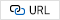28
A. Raha, H. Jayakumar, and V. Raghunathan, "Input-based Dynamic Reconfiguration of Approximate Arithmetic Units for Video Encoding", IEEE Transactions on Very Large Scale Integration (VLSI) Systems, Vol. 24, No. 3, pp. 846-857, Mar., 2016.29
H. Jiang, F. J. H. Santiago, H. Mo, L. Liu, and J. Han, “Approximate Arithmetic Circuits: A Survey, Characterization, and Recent Applications,” Proceedings of the IEEE, Vol. 108, No. 12, pp. 2108-2135, Dec., 2020.30
H. R. Myler and A. R. Weeks, The Pocket Handbook of Image Processing Algorithms in C, Prentice-Hall, Inc., USA, 1993.Hyoju Seo received her B.S and M.S. degrees at the School of Computer Science and Engineering from Kyungpook National University, Daegu, Republic of Korea, in 2020 and 2022, respectively, where she is currently pursuing a Ph.D. Her research interests include approximate computing, neuromorphic computing, deep learning accelerator, and image processing.

Hyelin Seok received a B.S. degree from the School of Computer Science and Engineering, Kyung-pook National University, Daegu, Republic of Korea in 2022, where she is pursuing an M.S. degree. Her research interests include computer architecture, approximate arithmetic, and new computing systems.

Jungwon Lee received a B.S. degree from the School of Computer Science and Engineeraaing, Kyung-pook National University, Daegu, Republic of Korea in 2021, where she is pursuing an M.S. degree. Her research interests include deep learning, approximate arithmetic, and approximate DRAM.

Youngsun Han received his B.S. and Ph.D. degrees in Electrical Engi-neering from Korea University, Seoul, South Korea, in 2003 and 2009, respectively. He was a senior engineer at the System LSI, Samsung Electronics, Suwon, South Korea, from 2009 to 2011. He was an assistant/associate professor with the Department of Electronic Engineering, Kyungil University, Gyeongsan-si, South Korea, from 2011 to 2019. He is currently an associate professor with the Department of Computer Engineering, Pukyong National University, Busan, South Korea. His research interests include quantum computing, high-performance computing, compiler construction, and microarchitecture.

Yongtae Kim received B.S. and M.S. degrees in electrical engineering from the Korea University, Seoul, Republic of Korea, in 2007 and 2009, respectively and a Ph.D. degree from the Department of Electrical and Computer Engineering from the Texas A&M University, College Station, TX, in 2013. From 2013 to 2018, he was a software engineer with Intel Corporation, Santa Clara, CA. Since 2018, he has been with the School of Computer Science and Engineering at Kyungpook National University, Daegu, South Korea, where he is currently an assistant professor. His research interests are in energy efficient integrated circuits and systems, particularly, neuromorphic computing and approximate computing, and new memory devices and architectures.